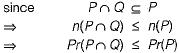Courses

# Test: Probability- 1

## 10 Questions MCQ Test Question Bank for GATE Computer Science Engineering | Test: Probability- 1

Description
This mock test of Test: Probability- 1 for GATE helps you for every GATE entrance exam. This contains 10 Multiple Choice Questions for GATE Test: Probability- 1 (mcq) to study with solutions a complete question bank. The solved questions answers in this Test: Probability- 1 quiz give you a good mix of easy questions and tough questions. GATE students definitely take this Test: Probability- 1 exercise for a better result in the exam. You can find other Test: Probability- 1 extra questions, long questions & short questions for GATE on EduRev as well by searching above.
QUESTION: 1

### Let A and B be any two arbitrary events, then, which one of the following is true?

Solution: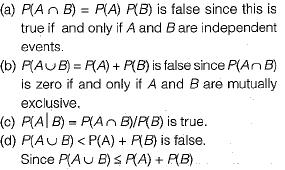QUESTION: 2

### A bag contains 10 white balls and 15 black balls. Two balls are drawn in succession. The probability that one of them is black and the other is white is

Solution: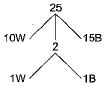Bag contains 10 white balls and 15 black balls. Required probability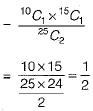QUESTION: 3

### Two dice are thrown simultaneously. The probability that at least one of them will have 6 facing up is

Solution:

P(atleast one of dice will have 6 facing
= 1 - P(none of dice have 6 facing up)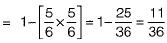QUESTION: 4

The probability that top and bottom cards of a randomly shuffled deck are both aces is-

Solution:

The probability that the bottom card of a randomly shuffled deck is ace = 4/52 because there are 4 aces out of total 52 cards.
From the remaining 3 aces out of SVcards the probability that the top card is also an ace = 3/51.
So required probability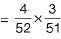QUESTION: 5

E1 and E2 are events in a probability space satisfying the following constraints: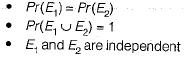The value of Pr(E1), the probability of the event E1, is

Solution:

Constraints are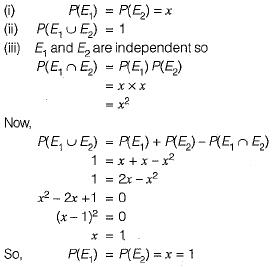QUESTION: 6

A program consists of two modules executed sequentially. Let f1(t) and f2(t) respectively denote the probability density functions of time taken to execute the two modules. The probability density function of the overall time taken to execute the program is given by

Solution:

Let the time taken for first and second modules be represented by x and y and total time = t.
∴ t = x + y is a random variable.
Now the joint density function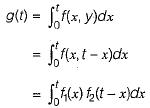which is also called as convolution of f1 and f2, abbreviated as f1 * f2.

QUESTION: 7

A sample space has two events A and B such that probabilities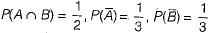What is P(A ∪ B)?

Solution:

We know that: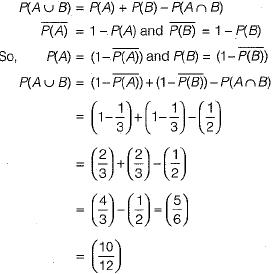QUESTION: 8

If the difference between the expectation of the square of a random variable (E[x2] and the square of the expectation of the random variable (E[x])2 is denoted by R, then

Solution: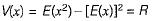where V(x) is the variance of x,
Since variance isand hence never negative,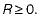QUESTION: 9

Consider a random variable Xthat takes values +1 and -1 with probability 0.5 each. The values of the cumulative distribution function F(x) at x = -1 and +1 are

Solution:

The p.d.t of the random variable is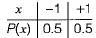The cumulative distribution function F(x) is the probability uptox as given below: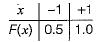QUESTION: 10

If P and Q are two random events, then the following is TRUE

Solution:

(a) is false since of Pand Q are independent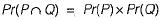which need not be zero.
(b) is false since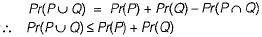(c) is false since independence and mutually exclusion are unrelated properties.
(d) is true